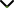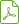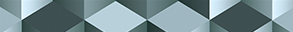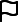# Recent Advance in Function Spaces and their Applications in Fractional Differential Equations

Publishing date
01 Feb 2019
Status
Published
14 Sep 2018

Guest Editors

1Curtin University of Technology, Perth, Australia

2Qufu Normal University, Qufu, China

# Recent Advance in Function Spaces and their Applications in Fractional Differential Equations

## DescriptionFractional differential equation is a new research area of analytical mathematics which provides useful tools to model many physical and biological phenomena and optimal control of complex processes with memory effects. Function space theory has played an important role in the study of various fractional differential equations and complex real world problems. Therefore, by using function space theory, understanding the characteristics of solutions and developing the properties of approximate solutions of this type of equations would have profound impact on many disciplines. New advancement of function space theory will greatly promote the development of fractional calculus theory, functional theory, and mathematical physics as well as their applications in differential and integral equations. The aim of this special issue is to report and promote the latest achievements and recent development in the well-posedness analysis and computational methods and function space theory for solving various fractional differential equations.

We invite researchers to submit original research articles as well as review articles on the recent development in the theory of function space and the applications of nonlinear fractional differential equations to sciences, technologies, and engineering.

Potential topics include but are not limited to the following:

• Function space theory including fractional derivative
• Boundary value problems of fractional differential equations
• Initial value problems of fractional differential equations
• Inequalities of fractional integrals and fractional derivatives
• Singular and impulsive fractional differential and integral equations
• Analysis and control in fractional differential equations
• Numerical analysis and algorithm for fractional differential equations
• Fixed point theory and application in fractional calculus
• Operator theory in function spaces and operator algebras
• Fractional functional equations in function spacesDownload Special IssueJournal metrics
Acceptance rate28%
Submission to final decision43 days
Acceptance to publication37 days
CiteScore2.000
Impact Factor1.896Author guidelinesEditorial boardDatabases and indexingSign up for content alerts

We are committed to sharing findings related to COVID-19 as quickly as possible. We will be providing unlimited waivers of publication charges for accepted research articles as well as case reports and case series related to COVID-19. Review articles are excluded from this waiver policy. Sign up here as a reviewer to help fast-track new submissions.